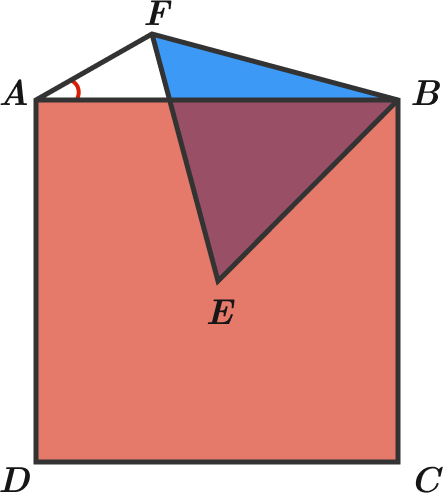# Vertices and angles

Geometry Level 3$ABCD$ is a square and $E$ is its center. $F$ is chosen in order to create an equilateral triangle with $B$ and $E.$ What is the measure (in degrees) of $\angle FAB?$

×

Problem Loading...

Note Loading...

Set Loading...